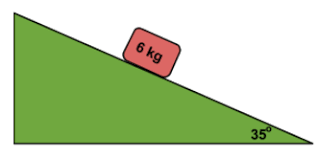• # 1.3 and 1.4 Quiz

Posted by Craig Messerman on 9/16/2021

LM3

a. Which of these students seems to have been the best at timing an event? Why?

b. Which one was the least consistent? Why?

1. 3.4, 3.7, 3.5, 3.5

2. 3.2, 3.6, 3.4, 3.4

3. 3.5, 3.0, 3.4, 3.3

LM4 (show all work and units!)

a. Calculate the velocity of a runner who runs 100 meters in 11.00 seconds, on our track.

b. How many minutes does it take to drive 50.0 km at a speed of 90.0 km/hr?

• # 5.4 Quiz

Posted by Craig Messerman on 5/10/2021

1. Calculate two frequencies that will resonate in a 0.50 m tube that is closed on one end.

2. Determine the frequency of this note that is resonating in this tube open at both ends. How long is the tube if 645 Hz resonates?

• # 5.2 Quiz

Posted by Craig Messerman on 5/4/2021

1. If the temp is 22 deg C, how long will it take for sound to echo off the end of a 1.5 m long tube?

• # 5.1 quiz

Posted by Craig Messerman on 4/28/2021

a. If a standing wave in a spring oscillates 10 times in 3.0 seconds what is the frequency of the wave?

b. What is the period of one oscillation?

c. If the wavelength of that wave is 4.0 meter, what is the speed of the wave through the spring?

d. What is the wavelength of the microwaves used in your cell phone radio to connect to the cell tower? The speed of light (electromagnetic waves) is 3.0 x 10^8 m/s, and the frequency of some cell phones is 2.1 GHz.

• # 4.1 quiz

Posted by Craig Messerman on 2/26/2021

1. How does a generator use magnets to make electricity?

2. With copper being non-magnetic, how can a copper pipe slow down a magnet?

• # LM 4.5 quiz

Posted by Craig Messerman on 9/2/2020

1. What is the average speed of a 500 mi race that takes 3 hours to finish?

2. What is the average velocity of that race (on an oval track)?

3. How far will a runner run at 4.0 m/s in 10 seconds?

4. How long does it take a runner to run 100 meters at 9.0 meters per second?

5. What is the acceleration of a car in m/s^2 if it goes from 0-60 miles per hour in 8 seconds (like my Rabbit!)

• # 2D #2 Quiz

Posted by Craig Messerman on 1/8/2020

a. If the projectile in the #1 quiz landed 5.0 meters away, what was Vi?

• # 2D#1 quiz

Posted by Craig Messerman on 1/6/2020

a. If a projectile is airborn for 1.5 seconds, how high did it fly? (Assume launch and landing at equal height, and no friction)

• # FOR 8 and 11 Quiz

Posted by Craig Messerman on 11/18/2019

If this box is accelerating down the ramp at 1.7 m/s/s, what is the coefficient of sliding friction between the box and ramp?• # FOR 4 Quiz

Posted by Craig Messerman on 10/28/2019

a. Show with a labelled free-body diagram the forces acting on this 60.0 kg skier (weight, force parallel to slope, force normal to slope)